# 2016PUPC普林斯顿大学物理竞赛Onsite答案免费下载

### Onsite现场竞赛部分### 2016PUPC普林斯顿大学物理竞赛完整版真题免费下载

Onsite现场竞赛部分此卷共3题

### 2016PUPC普林斯顿大学物理竞赛完整版答案免费下载

Problem 1. A Jelly-Filled Universe (10 points total)

a) With no other external forces on the particle, a = 0. We can show this using Newton’s second law:

- ka = ma,

which yields a = 0. Therefore,

x = x0 + v0t.

b) Again, using Newton’s laws we have F - ka = ma, which yields a = F/(k + m). We see that the pseudo-drag force acts by contributing an effective mass to the particle. Integrating, we getso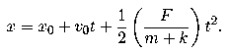c) We know that the net force on the particle is constant. Deﬁning the acceleration a0 = F/(k + m) from part b, we find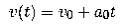The net work done is therefore the change in kinetic energy during the time T :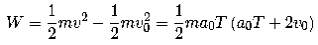d)Kinetic friction is a constant braking force, so in a given time interval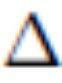t the particle experiences a decrease in speed ofv α-t. That is, the speed decreases at a constant rate. For viscous drag, the force increases linearly with speed, sov α-vt, which implies that the speed decreases exponentially fast v∼exp( -kt). Unlike the other forces, the pseudo-drag force only acts in the presence of other forces. Alone, it has no effect on the system. In the presence of other forces, it acts by increasing the effective mass of the particle to m →m + k.

e)The force of gravity is Fg = −mg, where m is the particle’s gravitational mass. Using Newton’s second law, we find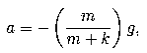so the integrated equations give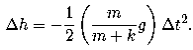Solving for the time elapsed, we findf)  It is indeed possible to replace g with an e↵ective gravitational ﬁeld ge↵. Studying Newton’s second law, we see that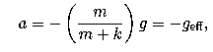which implies that we should set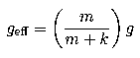Taking the limits, we getThese results make sense. As m→0, the particle becomes massless, so it is no longer affected by gravity. So, it doesn’t matter whether there is a gravitational field (g ≠ 0) or not (geff= 0). In the second case, we have that the particle is infinitely massive. We no longer expect the the pseudo-force, which contributes a constant, finite term k to the effective mass, to matter. Thus, we expect geff= g, since the pseudo-drag force no longer contributes.